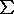## Largest planar graph with positive combinatorial curvature

Let G be a connected graph that is 2-cell embedded in some surface. For a vertex v and a face f of G, let deg(v) denote the degree of v and let deg(f) denote the size of f. We define the combinatorial curvature of the vertex v to be

f(v) = 1 - deg(v)/2 +1/deg(f),

where the sum is taken over all faces f incident with v (counting possible multiple incidences). This definition of curvature is rather natural and has been independently introduced by many authors (see, e.g., [1,2,3]). Higuchi  conjectured that if G is embedded in the plane such that f(v) > 0 for every vertex v, then G is finite. This conjecture was settled in  (in a more general form), where it was also shown that such a graph G usually cannot have too many vertices.

Theorem 1 (): Let G be a connected graph embedded in the plane so that every vertex and every face have degree at least 3. If f(v) is everywhere positive, then G is finite. Furthermore, if G is neither a prism, nor an antiprism, then G has at most 3444 vertices.

It would be interesting to find the smallest number C which could take the place of 3444 in the above theorem. The best lower bound we know of comes from the great rhombic icosidodecahedron. This polyhedron has 120 vertices, each of which is incident with one square, one hexagon, and one decagon. Thus, C is between 120 and 3444. Although little effort has been made in  to optimize the upper bound, it seems that a considerably more detailed analysis would be required to get close to the correct value for C.

Problem 1 (DeVos and Mohar): Let G be a connected graph embedded in the plane so that every vertex and face has degree at least 3. If f(v) is everywhere positive and G is neither a prism nor an antiprism, is it true that G has at most 120 vertices?

*Problem 1 has been answered in the negative by Tamás Réti et al.  who found an example based on the great rhombic icosidodecahedron with 138 vertices. Thus, we are posing a relaxed problem:

Problem 1* (DeVos and Mohar): Find the smallest integer N such that every connected graph G embedded in the plane so that every vertex and face has degree at least 3, whose combinatorial curvature f(v) is everywhere positive and G is neither a prism nor an antiprism, has at most N vertices.

References:

 M. DeVos and B. Mohar, An analogue of the Descartes-Euler formula for infinite graphs and Higuchi's conjecture, submitted in 2004.

 M. Gromov, Hyperbolic groups, in Essays in Group Theory, S. M. Gersten (Editor), M.S.R.I. Publ. 8, Springer, 1987, pp.75-263.

 Y. Higuchi, Combinatorial curvature for planar graphs, J. Graph Theory 38 (2001) 220-229.

 T. Réti, E. Bitay and Zs. Kosztolányi, On the Polyhedral Graphs with Positive Combinatorial Curvature, preprint, 2005.